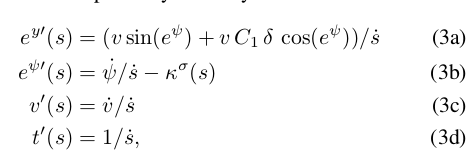# Time vs spatial dynamic model in race car example

Hi there,
First of all, thank you a lot for excellent tool. Early I used the ACADO and I was so happy when I found the ACADOSI noticed that ‘race_cars’ example is very similar a model from this paper
However there is a difference: in the paper all state variable are path dependent meanwhile in the example all state variables are time dependent.
Namely, all ODE eqations are divided on the velocity along a path (s_dot).However, in the race_cars example we don’t it
f_expl = vertcat(
sdota,
v * sin(alpha + C1 * delta),
v * C2 * delta - kapparef_s(s) * sdota,
Fxd / m * cos(C1 * delta),
derD,
derDelta,
)

So I have couple questions.
Which advantages does the time dependent model?
How to set different track boundaries along path in the case time dependent model?

Regards,
Alex